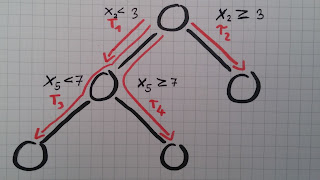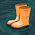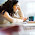## Saturday, October 3, 2015

### RuleFit: When disassembled trees meet Lasso

The RuleFit algorithm from Friedman and Propescu is an interesting regression and classification approach that uses decision rules in a linear model.

RuleFit is not a completely new idea, but it combines a bunch of algorithms in a clever way. RuleFit consists of two components: The first component produces "rules" and the second component fits a linear model with these rules as input (hence the name "RuleFit"). The cool thing about the algorithm is that the produced model is highly interpretable, because the decision rules have an easy understandable format, but you still have a flexible enough approach to capture complex interactions and get a good fit.

### Part I: Generate rules

The rules that the algorithm generates have a simple form:

if  x2 < 3  and  x5 < 7  then  1  else  0

The rules are generated from the covariates matrix X. You can also see the rules simply as new features based on your original features.

The RuleFit paper uses the Boston housing data as example: The goal is to predict the median house value in the Boston neighborhood. One of the rules that is generated by RuleFit is:

if  (number of rooms > 6.64)  and  (concentration of nitric oxide < 0.67) then 1 else 0

The interesting part is how those rules are generated: They are derived from Decision Trees, by basically ripping them apart. Every path in a tree can be turned into a decision rule. You simply chain the binary decisions that lead to a certain node et voilà, you have a rule. It is desirable to generate a lot of diverse and meaningful rules. Gradient boosting is used to fit an ensemble of decision trees (by regressing/classifying y with your original features X). Each resulting trees is turned into multiple rules.4 rules can be generated from a tree with 3 terminal nodes

Another way to see this step is a black box, that generates a new set of features X' out of your original features X. Those features are binary and can represent quite complex interactions of your original X. The rules are chosen to maximise the prediction/classification task at hand.

### Part II: Fit the rules

You will get A LOT of rules from the first step (and that is what you want). Since the first step is only a feature transformation function on your original data set you are still not done with fitting a model and also you want to reduce the number of rules. Lasso or L1 regularised regression is good in this scenario. Next to the rules also all numeric variables from your original data set will be used in the Lasso linear model. Every rule and numeric variable gets a coefficient (beta). And thanks to the regularisation, a lot of those betas will be estimated to zero. The numeric variables are added because trees suck at representing simple linear relationships between y and x. The outcome is a linear model that has linear effects for all of the numeric variables and also linear terms for the rules.

The paper not only introduces RuleFit and evaluates it, but it also comes with a bunch of useful tools, (comparable to Random Forest): Measurement tools for variable importance, degree of relevance of original input variables and interaction effects between variables.

There are currently two implementations out there one from the authors in R and one in the TMVA toolkit.

tl;dr  The RuleFit regression and classification algorithm works by boosting an ensemble of decision trees, creating decision rules from those trees and throwing the rules (+ the numeric covariables) at L1 regularized regression (Lasso). Try it out.

1.This looks interesting. Any thoughts on semi automatically turning a random forest into an ensemble of rule fit models and what the performance would be like? Seems like a natural fit to predicting continues variables.

1.It is possible, but I don't know what is better performance wise. Instead of generating the trees with Gradient boosting, you can also generate the trees from Random Forests. In fact, the TMVA toolkit implements both using Gradient boosting or Random Forests for rule generation. From one Random Forest you generate all the rules and put them into Lasso.

2.Hello,
In our project we've combined your python implementation for RuleFit. While this method works very well (R^2 ranges from 0.75 to 0.85) on our test group, the code outputs 10k+ rules in excel spreadsheet, all of which have a coeff of '0'.

More over, out of these 10k+ rules, we did not see any indication to linear rules (i.e. always apply). In order to test the validity of the model in our project, we wish to attain 'X' most important rules and their respective coeff and predict the "Y expected" manually.

Thanks for your help, we would very much like to also send our data to you so perhaps you'll see the issues for yourself.

Will it be possible to do so?

With much regards,

Solar-Cell Research group

1.Hello,

thank you for your comment. I am glad someone is finding my implementation useful. Please be aware that the code is not used and tested thoroughly, so it might have bugs.

Thanks to your input I made some necessary changes to the get_rules() method: Now it also outputs the coefficients for the linear terms and there is a 'type' column to distinguish linear and rule terms. Before the change, the linear terms were excluded. Also rules with an estimated coefficient of 0 are now filtered out by default.

3.### Explaining the decisions of machine learning algorithms

Being both statistician and machine learning practitioner, I have always been interested in combining the predictive power of (black box) ma...